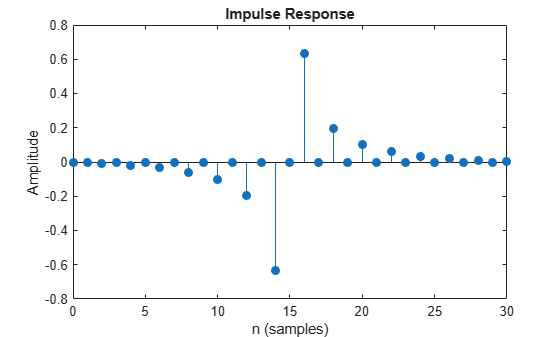firtype

Determine type (1-4) of linear phase FIR filter System object

Description

example

type = firtype(sysobj) determines the type (1 through 4) of the FIR filter System object™

For more input options, see firtype in Signal Processing Toolbox™.

Examples

collapse all

Design a Hilbert transformer of order 30 with a transition width of 0.2π rad/sample. Use least-squares minimization to obtain an equiripple linear-phase FIR filter. Plot the zero-phase response in the interval [–π,π).

d = fdesign.hilbert('N,TW',30,0.2);
Hd = design(d,'equiripple','SystemObject',true);
zerophase(Hd,'whole')The impulse response of this even-order type-3 filter is antisymmetric.

impz(Hd)ftype = firtype(Hd)
ftype = 3

Design a minimum-order Hilbert transformer that has a sample rate of 1 kHz. Specify the width of the transition region as 10 Hz and the passband ripple as 1 dB. Display the zero-phase response of the filter.

fs = 1e3;
d = fdesign.hilbert('TW,Ap',10,1,fs);
hd = design(d,'equiripple','SystemObject',true);
zerophase(hd,-fs/2:0.1:fs/2,fs)Input Arguments

collapse all

Input FIR filter with real and linear phase, specified as one of the following filter System objects:

To check if a filter has linear phase, use the islinphase function. To check if a filter has real coefficients, use the isreal function.

Output Arguments

collapse all

FIR filter type, defined as one of the following:

1 –– Type 1 filter with even order symmetric coefficients.

2 –– Type 2 filter with odd order symmetric coefficients.

3 –– Type 3 filter with even order antisymmetric coefficients.

4 –– Type 4 filter with odd order antisymmetric coefficients.